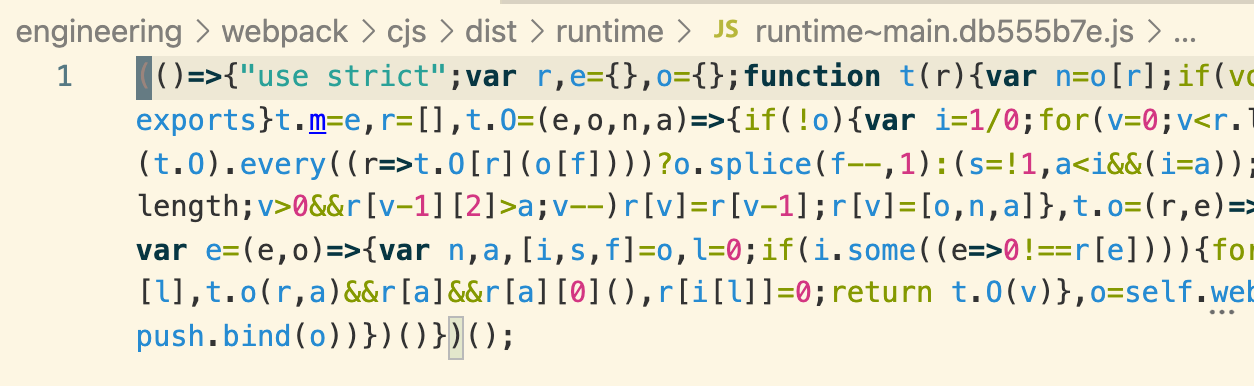# # javascript 代码是如何被压缩的

`jquery` 时代，当我们引用它的 CDN 地址时，在线上需要引用的是 `jquery.min.js`## # 如何压缩代码体积？

### # 去除多余字符: 空格，换行及注释

``````// 对两个数求和
function sum (a, b) {
return a + b;
}
``````

``````function sum(a,b){return a+b}
``````

### # 压缩变量名：变量名，函数名及属性名

``````function sum (first, second) {
return first + second;
}
``````

``````// 压缩: 缩短变量名
function sum (x, y) {
return x + y;
}

// 再压缩: 去除空余字符
function s(x,y){return x+y}
``````

### # 解析程序逻辑：合并声明以及布尔值简化

``````// 压缩前
const a = 3;
const b = 4;

// 压缩后
const a = 3, b = 4;
``````

``````// 压缩前
!b && !c && !d && !e

// 压缩后
!(b||c||d||e)
``````

### # 解析程序逻辑: 编译预计算

``````// 压缩前
const ONE_YEAR = 365 * 24 * 60 * 60

// 压缩后
const ONE_YAAR = 31536000
``````

``````// 压缩前
function hello () {
console.log('hello, world')
}

hello()

// 压缩后
console.log('hello, world')
``````

## # AST

`AST`，抽象语法树，js 代码解析后的最小词法单元，而这个过程就是通过 Parser 来完成的。

• eslint: 校验你的代码风格
• babel: 编译代码到 ES 低版本
• taro/mpvue: 各种可以多端运行的小程序框架
• GraphQL: 解析客户端查询## # uglify、terser 与 swc

`webpack` 中内置的代码压缩插件就是使用了 `terser`，看一段它工作的代码:

``````const { minify } = require('terser')
const code = 'function add(first, second) { return first + second; }'
const result = await minify(code, { sourceMap: true })
console.log(result.code)
console.log(result.map)
``````

``````{
{
ecma: 8,
},
compress: {
ecma: 5,
warnings: false,
comparisons: false,
inline: 2,
},
output: {
ecma: 5,
ascii_only: true,
}
}
``````

## # 在 webpack 中压缩代码

``````optimization: {
minimize: isEnvProduction,
minimizer: [
new TerserPlugin({
terserOptions: {
parse: {
ecma: 8,
},
compress: {
ecma: 5,
warnings: false,
comparisons: false,
inline: 2,
},
output: {
ecma: 5,
ascii_only: true,
},
},
sourceMap: true
})
]
}
``````

## 关于山月

Last Updated: 7/21/2019, 11:25:08 AM